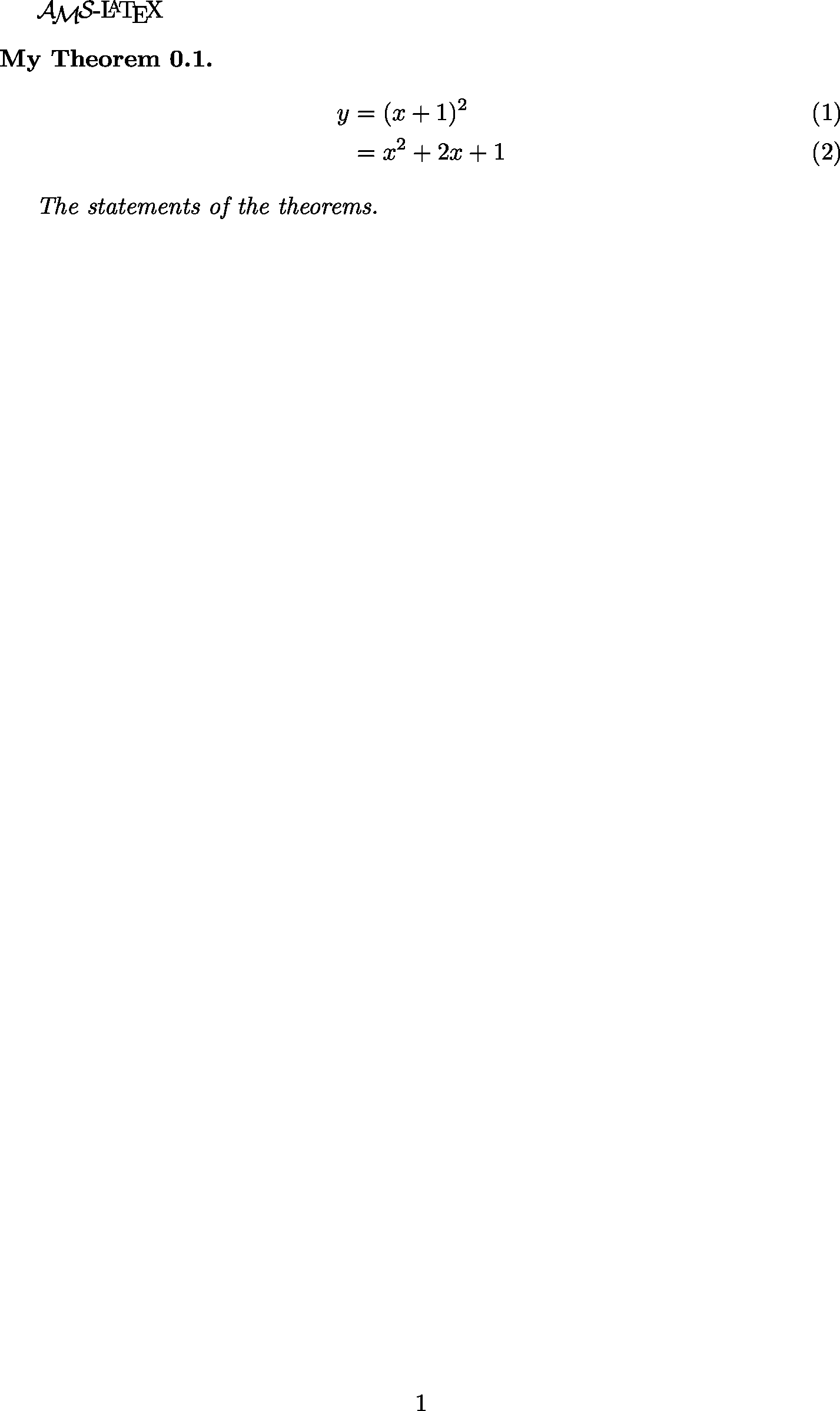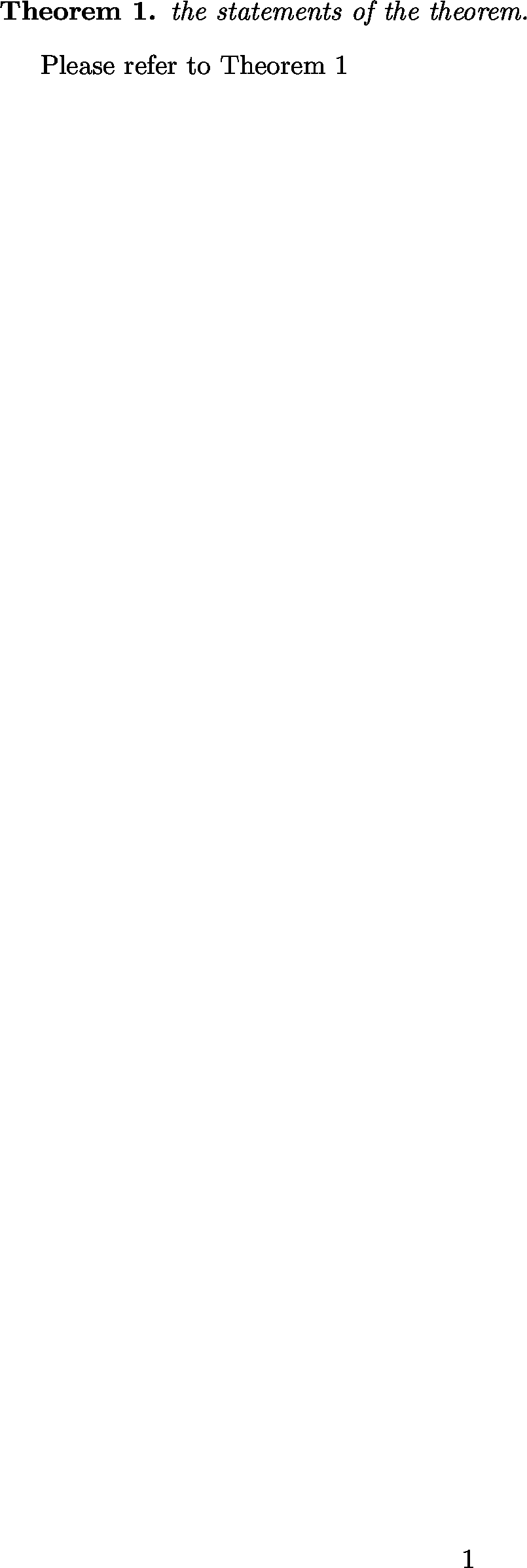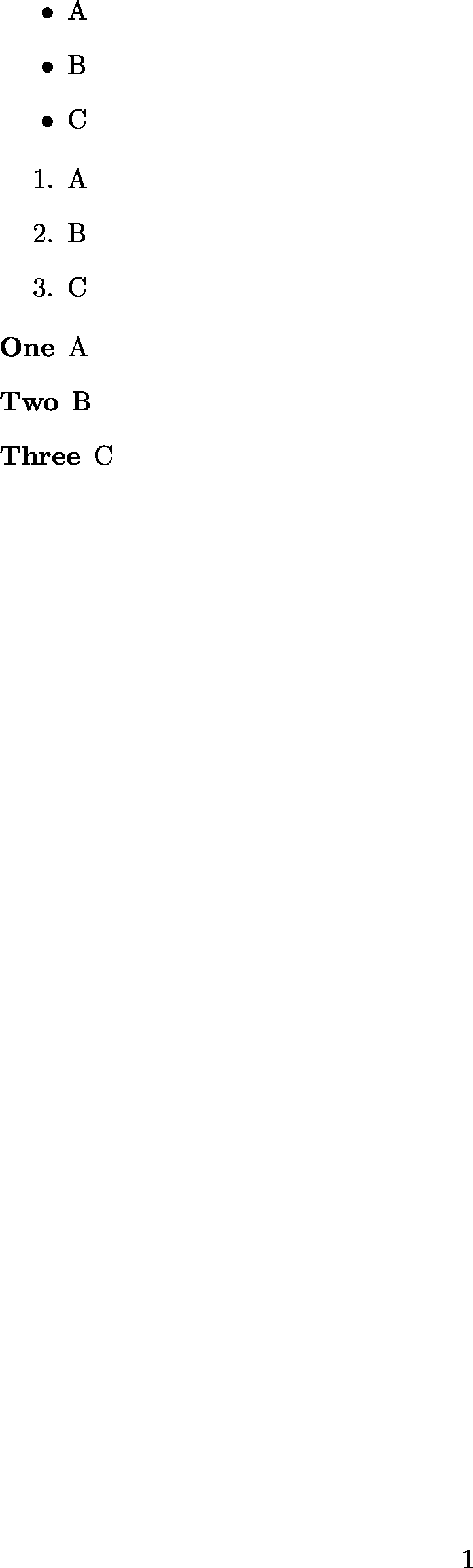# LaTeX 教學¶

## 基本語法¶

### 範例一 - 非中文內容¶

\documentclass{article}
\begin{document}

% 以下撰寫內容

Hello World

% 以上撰寫內容

\end{document}


$pdflatex hello.tex # 產生 hello.pdf  轉成圖片： # PNG$ latex2png hello.tex    # 產生 hello.png

# TODO: SVG


### 範例二 - 稍微完整一點的架構¶

\documentclass{article}

% 設定區域

\title{文章標題}
\author{作者}

\begin{document}

% 以下撰寫內容

Hello World

% 以上撰寫內容

\end{document}


### 範例三 - 數學式子¶



\documentclass{article}

% 設定區域

\usepackage{xeCJK}
\setCJKmainfont{Noto Sans CJK TC}

\title{文章標題}
\author{作者}

\begin{document}

% 以下撰寫內容

% 以上撰寫內容

\end{document}




### 範例七 - AMS-LaTeX¶

AMS-LaTeX 是由美國數學學會（American Mathematical Society）開發的套件， 提供了許多數學相關的支援。

• amssymb：提供原本 LaTeX 沒有的符號

• amsamth：提供 align 等方便的環境

• amsthm：提供比較好使用定理的環境

\usepackage{amssymb, ammath, amsthm}


AMS-LaTeX 也提供一種 Document Class 可以使用， 使用時會自動 amsmath、amsthm 和部份 amssymb， 如果要全套 amssymb 還是需要自己讀入， 使用範例：

\documentclass{amsstart}


% 定義定理環境 thm
% 顯示時標示為 Theorem
\newtheorem{thm}{Theorem}

\begin{thm}
The statements of the theorem.
\end{thm}


\newtheorem{thm}{Theorem}[section]


\documentclass{article}

% 設定區域

\usepackage{amssymb, amsmath, amsthm}

\title{文章標題}
\author{作者}

\newtheorem{thm}{My Theorem}[section]

\begin{document}

% 以下撰寫內容

\AmS-\LaTeX

\begin{thm}
\begin{align}
y &= (x+1)^2 \\
&= x^2+2x+1
\end{align}

The statements of the theorems.
\end{thm}

% 以上撰寫內容

\end{document}\newtheorem{thm}{My Theorem}
\newtheorem{lem}[thm]{My Lemma}


\documentclass{article}

% 設定區域

\usepackage{amssymb, amsmath, amsthm}

\title{文章標題}
\author{作者}

\newtheorem{thm}{My Theorem}
\newtheorem{lem}[thm]{My Lemma}

\begin{document}

% 以下撰寫內容

\AmS-\LaTeX

\begin{thm}
\begin{align}
y &= (x+1)^2 \\
&= x^2+2x+1
\end{align}

The statements of the theorems.
\end{thm}

\begin{thm}
\begin{align}
y &= (x+1)^2 + 1
\end{align}
\end{thm}

\begin{thm}
\begin{align}
y &= (x+1)^2 + 2
\end{align}
\end{thm}

\begin{lem}
statement of lemma
\end{lem}

\begin{lem}
another statement of lemma
\end{lem}

% 以上撰寫內容

\end{document}\newtheorem*{mainthm}{Main Theorem}


amsthm 還支援不同的定理風格， 包含：

• plain

• definition

• remark

\theoremstyle{plain}
\newtheorem{thm}{Theorem}

\theoremstyle{definition}
\newtheorem{def}{Definition}

\theoremstyle{remark}
\newtheorem{rmk}{Remark}


\documentclass{article}

% 設定區域

\usepackage{amssymb, amsmath, amsthm}

\theoremstyle{plain}
\newtheorem{thm}{Theorem}

\theoremstyle{definition}
\newtheorem{defn}{Definition}

\theoremstyle{remark}
\newtheorem{rmk}{Remark}

\begin{document}

% 以下撰寫內容

\begin{thm}
The statements of the theorems.
\end{thm}

\begin{defn}
The statements of the definition.
\end{defn}

\begin{rmk}
The statements of the remarks.
\end{rmk}

% 以上撰寫內容

\end{document}


\begin{thm}\label{T:major}
the statements of the theorem.
\end{thm}

% 引用

Please refer to
Theorem ~\ref{T:major}


\documentclass{article}

% 設定區域

\usepackage{amssymb, amsmath, amsthm}

\newtheorem{thm}{Theorem}

\begin{document}

% 以下撰寫內容

\begin{thm}\label{T:major}
the statements of the theorem.
\end{thm}

% 引用

Please refer to
Theorem~\ref{T:major}

% 以上撰寫內容

\end{document}### 範例八 - 嵌入圖片¶

\usepackage{graphicx}


\includegraphics[width=5cm]{pic.png}


\begin{center}
\includegraphics[width=5cm]{pic.png}
\end{center}


\begin{figure}
\begin{center}
\includegraphics[width=5cm]{pic.png}
\end{center}
\caption{Description}
\end{figure}


• h：Here，這裡

• t：Top，頂端

• b：Bottom，底端

• p：Page，本頁

\begin{figure}[h]
...
\end{figure}


\caption{Description}\label{My Pic}

Here is the picture ~\ref{My Pic}


\documentclass{article}

% 設定區域

\usepackage{graphicx}

\begin{document}

% 以下撰寫內容

\begin{figure}
\begin{center}
\includegraphics[width=5cm]{rust.png}
\end{center}
\caption{Description}
\label{fig:rust}
\end{figure}

% 引用

See the figure~\ref{fig:rust} on page~\pageref{fig:rust}

% 以上撰寫內容

\end{document}


### 範例九 - 列表¶

% 沒有編號

\begin{itemize}
\item A
\item B
\item C
\end{itemize}

% 有編號

\begin{enumerate}
\item A
\item B
\item C
\end{enumerate}

% 客製編號

\begin{description}
\item [One] A
\item [Two] B
\item [Three] C
\end{description}


\documentclass{article}

% 設定區域

\begin{document}

% 以下撰寫內容

% 沒有編號

\begin{itemize}
\item A
\item B
\item C
\end{itemize}

% 有編號

\begin{enumerate}
\item A
\item B
\item C
\end{enumerate}

% 客製編號

\begin{description}
\item [One] A
\item [Two] B
\item [Three] C
\end{description}

% 以上撰寫內容

\end{document}### 範例十 - 陣列和表格¶

$\begin{array}{ccc} 1 & 2 & 3 \\ 4 & 5 & 6 \\ 7 & 8 & 9 \end{array}$


• c：對齊中間

• l：對齊左邊

• r：對齊右邊

\begin{tabular}{ccc}
item1 & item2 & item3 \\
1 & 2 & 3 \\
4 & 5 & 6
\end{tabular}


\hline 加上橫線：

\begin{tabular}{ccc} \hline
item1 & item2 & item3 \\ \hline
1 & 2 & 3 \\ \hline
4 & 5 & 6 \\ \hline
\end{tabular}


\begin{tabular}{|c|c|c|} \hline
item1 & item2 & item3 \\ \hline
1 & 2 & 3 \\ \hline
4 & 5 & 6 \\ \hline
\end{tabular}


$\left( \begin{array}{ccc} 1 & 2 & 3 \\ 4 & 5 & 6 \\ 7 & 8 & 9 \end{array} \right)$


\left\right 一定要成對出現， 但是有時候我們只想要單邊， 下面是單邊的範例：

$|x| = \left\{ \begin{array}{rr} x, & \mbox{if x \geq 0} \\ -x, & \mbox{if x < 0} \end{array} \right.$


（我們可以用 \mbox 在數學式中輸入純文字）

### 可點的連結¶

\url{https://github.com/wdv4758h/notes/}